# Quantized Angular Momentum

In the process of solving the Schrodinger equation for the hydrogen atom, it is found that the orbital angular momentum is quantized according to the relationship: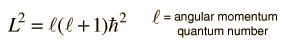It is a characteristic of angular momenta in quantum mechanics that the magnitude of the angular momentum in terms of the orbital quantum number is of the formand that the z-component of the angular momentum in terms of the magnetic quantum number takes the form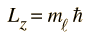This general form applies to orbital angular momentum, spin angular momentum, and the total angular momentum for an atomic system. The relationship between the magnitude of the angular momentum and its projection along any direction is space is often visualized in terms of a vector model.
Index

 HyperPhysics***** Quantum Physics R Nave
Go Back

# Orbital Angular Momentum

The orbital angular momentum of electrons in atoms associated with a given quantum state is found to be quantized in the form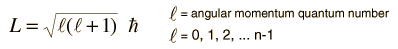This is the result of applying quantum theory to the orbit of the electron. The solution of the Schrodinger equation yields the angular momentum quantum number. Even in the case of the classical angular momentum of a particle in orbit,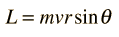the angular momentum is conserved. The Bohr theory proposed the quantization of the angular momentum in the form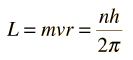and the subsequent application of the Schrodinger equation confirmed that form for the orbital angular momentum.

The spectroscopic notation used for characterizing energy levels of atomic electrons is based upon the orbital quantum number.

### Vector model of angular momentum

Index

 HyperPhysics***** Quantum Physics R Nave
Go Back

# Total Angular Momentum

When the orbital angular momentum and spin angular momentum are coupled, the total angular momentum is of the general form for quantized angular momentum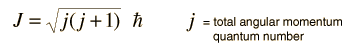where the total angular momentum quantum number is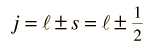This gives a z-component of angular momentum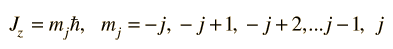This kind of coupling gives an even number of angular momentum levels, which is consistent with the multiplets seen in anomalous Zeeman effects such as that of sodium.

As long as external interactions are not extremely strong, the total angular momentum of an electron can be considered to be conserved and j is said to be a "good quantum number". This quantum number is used to characterize the splitting of atomic energy levels, such as the spin-orbit splitting which leads to the sodium doublet.

 Total angular momentum with multiple electrons
 Total angular momentum for rigid rotator
Index

 HyperPhysics***** Quantum Physics R Nave
Go Back